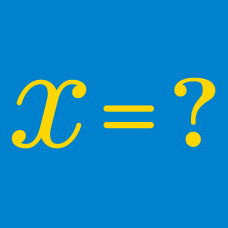Algebra

# Algebra Warmups: Level 1 Challenges

If $a = \sqrt b$ and $a = 3$, what is the value of $b$?

$\large \color{#624F41}5^{55} + \color{#624F41}5^{55} + \color{#624F41}5^{55} + \color{#624F41}5^{55} +\color{#624F41} 5^{55} = \, ?$

$\large \dfrac{ \color{#20A900}2^{\color{#3D99F6}{5}}\times \color{#20A900} 2^{\color{#3D99F6}{7}}}{\color{#20A900} 2^{{\color{#3D99F6}{10}}}\times \color{#20A900} 2^{\color{#3D99F6}{3}}}\times \color{#69047E}{3^0} = \ ?$

How many digits does the number $1000^{ 1000 }$ have?

Given that $x$ is a real number such that $x^2-4x+4=0$, find the value of $x$.

×